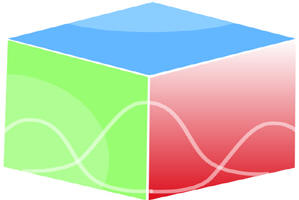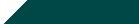``Home| Journals | Statistics Online Expert | About Us | Contact UsUntitled Document

[

Multi-valued Mappings in D-metric space

Bhosle Archana Venketesh1*, R.V. Kakde2

1Research Scholar, S.R.T.M.U. Nanded, Maharashtra, INDIA.

2Head, Department of Mathematics, Shri Shivaji College, Kandhar, Nanded, Maharashtra, INDIA.

Email:

Research Article

Abstract          Dhage introduced a new concept of D-metric space thereby which it has been possible to determine the geometrical nearness that is the distance between two or more points of the set under consideration. It is also now clear that the theory of D-metric spaces is much useful in the study of approximation theory. In this paper we give multi-valued mappings in D-metric space.

Key words: D-metric space, multi value mappings.

INTRODUCTION

Motivated by the measures of nearness between two or more objects with respect to a specific property or characteristic called the parameter of the nearness. Dhage  introduced a new concept of D-metric space there by which it has been possible to determine the geometrical nearness i.e. the distance between two or more points of the set under consideration. It is also now clear that the theory of D-metric spaces is much useful in the study approximation theory and various areas of mathematics.

DEFINITION 1.1

The notion of a D-metric space appeared in Dhage  is as follows:

Let X denotes a non-empty set and R+, the set of all non-negative real numbers. Then X together with a function D : X x X x X → R+ , is called a D-metric space if it satisfies the following properties :

1. D(x, y, z) = 0x = y = z               (coincidence)
2. D(x, y, z) = D(P{x, y, z})               (Symmetry)

P denotes the permutation function and

1. D(x, y, z) ≤ D(x, y, a) + D(x, a, z) + D(a, y, z)

For x, y, z, a  X                           (Tetrahedral inequality)

DEFINITION 1.2

A sequence {xn}  X is said to be convergent and converges to a point x if

(xm, xn, x) = 0

A sequence {xn}  X is called D-cauchy if

(xm, xn, xp) = 0

DEFINITION 1.3

A complete D-metric space is one in which every D-Cauchy sequence converges to a point in it.

DEFINITION 1.4

A set S  X is said to be bounded if there exist a constant M > 0, such that D(x, y, z) ≤ M for all x, y, z  S. and the constant M is called a D-bound of S.

It is known that the D-metric D is a continuous function on X3 in the topology of D-metric convergence which is Hausdorff in nature. Some specific examples of a D-metric space appeared in Dhage [2, 4] as follows:

EXAMPLE 1

Let X denote a non-empty set and define a function

D1 : X x X x X → [0, ∞) by

D1(x, y, z) = K max {d(x, y), d(y, z), d(z, x)}   For x, y, z  X.

Where K > 0 and d is an ordinary metric on X. Clearly the function D1 is a D-metric.

EXAMPLE 2

Let X denotes a non-empty set and defines a function

D2 : X x X x X → [0, ∞) by

D2(x, y, z) = d(x, y) + d(y, z) + d(z, x)      For x, y, z  X.

Where d is an ordinary metric on X. Clearly the function D2 is a D-metric on X and consequently (X, D2) is a D-metric space.

The Banach contraction mapping principle analogue proved by Dhage [1, 2] in a D-metric space is as follows :

THEOREM 1

Let X be a complete and bounded D-metric space and let f: X → X satisfy

D(fx, fy, fz) ≤ α D(x, y, z)                                                                                                               (1.1)

For all x, y, z  X and 0 ≤ α <1. Then F has a unique fixed point.

Let f : X → X then an orbit of the function f at a point x  X is a set O(x) is given by;

O(x) = {x, fx, f 2x, …….}                                                                                                                (1.2)

By O(x) we mean the closure of the orbit O(X), x  X in X. A D-metric space X is said to be f-orbitally bounded if the orbit O(X) is bounded for each x  X.

A D-metric space X is said to be f-orbitally complete if every D-cauchy sequence {xn}  O(x), x  X, converges to a point in X. A mapping f : X → X is called f-orbitally continuous if {xn}  O(x), x  X, xn → x* implies fxn → fx*.

The condition of theorem (1) under weaker condition obtained in a recent paper by Dhage and Rhoades  is as follows :

THEOREM 2

Let f be a self map of a f-orbitally complete D-metric space X satisfying (1.1). Then f has a unique fixed point x* and the sequence      {f nx}, x  X of successive interaction of f converges to x*.

Recently two more basic contraction mapping principles have been obtained by Dhage  for the self mappings f and g of a D-metric space X characterized by the inequalities :

D(fx, fy, z) ≤ α D(x, y, z)                                                                                                                            (2.1)

For all x, y   X and

Z   O(x)  O(y)

Where 0 ≤ α <1. And

D(fx, fy, z) ≤ α D(gx, gy, z)     For all x, y   X                                                                                           (2.2)

With some restriction on Z. and 0 ≤ α <1.

Obviously condition (2.1) does not necessarily imply condition (1.1) on a D-metric space X.

EXAMPLE 3

Let X = [0, 1]  {8} be equipped with the usual Euclidean metric |.| define a D-metric D on X by

D(x, y, z) = max {|x – y|, |y – z|, |z – x|}          For x, y, z  X

Consider the mapping f : X → X defined by

f(x) = {x/2 if x  [0, 1]

1                 otherwise

Now f satisfies condition (1.1) with α = ½ and has a unique fixed point x* = 0. But for x = 0, y =1 and z = 8 by (2.2) we get –

D(f(0), f(1), 8) = D(0,  8) ≠ 8 ≤ α8

= α D(0, 1, 8)

For any α < 1. This shows that the map f does not satisfy condition (2.2) on X.

DEFINITION 1.5

Let 2x and CB(X) denote the class of non-empty, closed and bounded subset of X respectively. A mapping T : X → 2x is called a multi-valued mapping on a D-metric space X. A point u  X is called a fixed point of T if u  Tu.

DEFINITION 1.6

A mapping T : X → 2x is continuous in a D-metric space X is for any sequence (xn) in X with xn → x as n → ∞, we have

= 0

A function G of a D-metric space X into non-negative reals is called T-orbitally lower semi continuous at a point P if (xn) is a sequence in O(x) and xn → P implies that G(P) ≤ lim inf G(xn)

DEFINITION 1.7

Let B(X, D) denote the family of all bounded subset of X for any sets A, B, C in B(X, D) we define Hausdorff D-metric denoted HD as under :

HD(A, B, C) = max {}

xεA                              xεB                                 xεC

Where,

D(X, B, C) = d(x, A) + d(A, B) + d(x, B)

Recall that,

D(X, A) = inf {d(x, y) : y  A} and

D(A, B) = inf {d(a, b) : a  A, b  B}

DEFINITION 1.8

Let (X, D) be a complete D-metric space and HD the Hausdorff D-metric on the family of all bounded subset of X.

Let CB(X, D) denote the family of all non-empty closed and bounded subset of X. The set valued mapping T is called Lipschitz mapping if for all x, y, z  X, there exists a constant K such that

HD (Tx, Ty, Tz) ≤ KD (x, y, z)

We say that T is a set-valued contraction if K < 1.

THEOREM 3

Let (X, D) be a complete D-metric space and CB(X, D) the family of all non-empty closed and bounded subsets of X with Hausdorff D-metric HD suppose that T : X → CB(X, D) is a set valued Lipschitz mapping then T has a fixed point.

PROOF

We select x0  X and xi  T, then by the definition of HD there exists x2  Tx1, and xm  Txm-1, such that ;

D(x1, x2, xm) ≤ HD (Tx0, Tx, Txm-1) + k

≤ k D (x0, x1, xm-1) + k                                                                                             (3.1)

Where m > n for all positive integers m, n.

Similarly there exists x3  Tx2, such that ;

D(x2, x3, xm-1) ≤ HD (Tx0, Tx1, Txm-2) + k

≤ k D (x0, x1, xm-2) + k

Again;

D(x1, x2, xm-1) ≤ HD (Tx0, Tx1, Txm-2) + k

≤ k D (x0, x1, xm-2) + k

Hence from (3.1) we have,

D(x2, x3, xm) ≤ k {kD(x0, x1, xm-2) + k} + k

≤ k2D(x0, x1, xm-2) + (k2 + k)

Repeating the argument for all n, we have;

D(xn, xn+1, xm) ≤ HD (Txn-1, Txn, Txm-1) + k

≤ D (xn-1, xn, xm-1) + k                                                                                                      (3.2)

Similarly;

D(xn-1, xn, xm-1) ≤ HD (Txn-2, Txn-1, Txm-2) + k

≤  D (xn-2, xn-1, xm-2) + k

Therefore from (3.2) we get

D(xn, xn+1, xm) ≤ k {kD(xn-2, xn-1, xm-2) + k} + k

≤ k2D(xn-2, xn-1, xm-2) + (k2 + k)                                                                                            (3.3)

Again;

D(xn-2, xn-1, xm) ≤ kD (xn-3, xn-2, xm-3) + k                                                                                                      (3.4)

Hence from (3.2), (3.3), (3.4) we get by induction on m –

D(xn, xn+1, xm-2) ≤ kD(xn-1, xn, xm-1) + k

≤ k2D(xn-2, xn-1, xm-2) + (k2 + k)

≤ k3D(xn-3, xn-2, xm-3) + (k3 + k2 + k)

:           :

:           :

≤ knD(x0, x1, xm-n) + (kn +… +k3 + k2 + k)

Thus;

D(xn, xn+1, xm-2) ≤ knD(x0, x1, xm-n) +

= knD(x0, x1, xm-n) + kn

= kn k + kn k’

= kn (k + k’)

Where; k = D(x0, x1, xm-n) > 0 and

k =  > 0

Are D-bounds of X. By Lemma it follows that (xn) is a D-Cauchy sequence. Since X is complete there exists x  X such that

Since T is continuous, we get

x =

= T  = Tx

Which shows that x is the fixed point of T.

REFERENCES

1. Dhage B.C.: A study for some fixed point theorems, Ph.D. thesis, Marathwada University, Aurangabad India (1984).
2. Dhage B.C.: Generalized metric spaces and mappings with fixed points. Bull Calcutta, Math. Soc. 84(4) (1992) Page No.329-336.
3. Dhage B.C.: Some topics in D-metric spaces and fixed point theory.
4. Dhage B.C.: Generalized metric spaces and topological structure I, Anale le. Stint, university, A1. L-cuza, Iasi, 44 (1998).
5. Dhage B.C. A.M. Pathan and B.E.Rhoads, A general existence Principle for fixed theorems in D-Metric Spaces. Internal J. Math and Math. Sci. Vol. 23.7 (2000), Page No. 441-448.
6. B.C. Dhage and B.E. Rhoades, A comparison of two contraction principles. Math Sci.Res. Hot line 3 (1999) Page No.49-53.
7. S.Nadler, Multi-valued contraction mappings, Pac. Jr. of Math. Vol.20, vol.27(1) (1968) Page No.192-194.
8. Dhage B.C.: on two basic contraction mapping principles in D-metric spaces. The East Asian Math community (1998)